# How to Add Total Row in an Excel Table [3 Quick Ways 2023]Written By Farhan Max

Inserting a total row in your Excel sheet will help you summarize the data. While managing a number-based dataset, you might often include a subtotal row at the end.

As Excel first introduced the Table feature in the 2007 version, some in-built features also help aggregate the data. And that too in tabular format. Furthermore, you can use the row for sum, average, count, minimum, maximum, and other sum functions.But, sometimes, adding a total row can split the Table format if not implemented properly.

I’ve shared the proper methods of including a total row in Excel to avoid that issue. Keep reading till the end of this article.

Check out our separate post on Highlight Duplicate Values in Excel

## How to Add a Total Row in Excel

Using keyboard shortcuts is the fastest way to add total rows in MS Excel. Select the range of rows you want to get a total result for; press Ctrl+Shift+T on your keyboard. You’ll notice a total row is added at the end of the selected rows.

You can follow further procedures if the shortcut method doesn’t work.

First, you need to use the table format to add rows. But it’s not mandatory. You can add rows without creating a table in Excel. We’ll further discuss the procedure of creating a total row without a design tab.

Now let’s get back to where we started.

Read more on how to Combine First and Last Names in MS Excel

Here are the methods to add total row in MS Excel:

### 1. Insert a Total Row from Table Design Tab

Adding the total row from the Excel Table design is the quickest method you’ll find. For that, you’ll need a table with your data. If the data aren’t included in a table, create a new one. Select your data to create a new table and navigate to Insert > Table.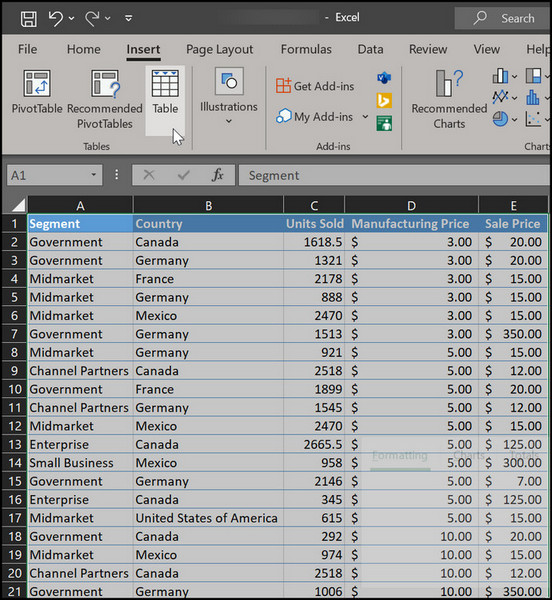Then proceed to the following steps to include a total row at the end.

Here are the steps to create a total row in Excel:

• Select the table you created.
• Move to the Table Design tab.
• Tick on the Total row checkbox from the Table Style Options group.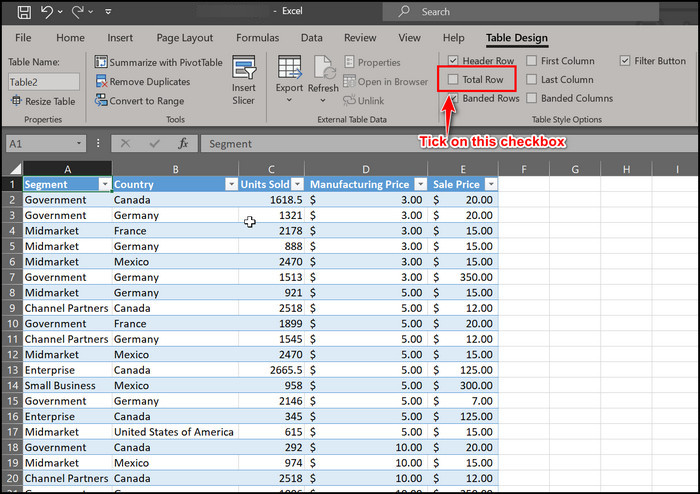When you tick on the total row from there, you’ll notice a new row named Total added to your table. By default, it will be sum total of the columns. But you can change it.

Click on the drop-down menu next to the fill handle, and you’ll find other options for the total row, such as average, count, count numbers, max, min, etc.

Also, check out our separate post on how to Install Macro in Excel

### 2. Use Context Menu to Insert Total Row

A context menu is a list of options that you can use to modify a cell. You can open this menu just by right-clicking any of the cells on your worksheet. Follow further instructions to add the total row via the context menu.

Here are the steps to use the context menu for adding a total row:

• Select all the cells that you want to total row for.
• Right-click on any of those cells. A long menu will pop up.
• Locate the Table from the list and move your mouse cursor on it.
• Select Totals Row from the sub-menu.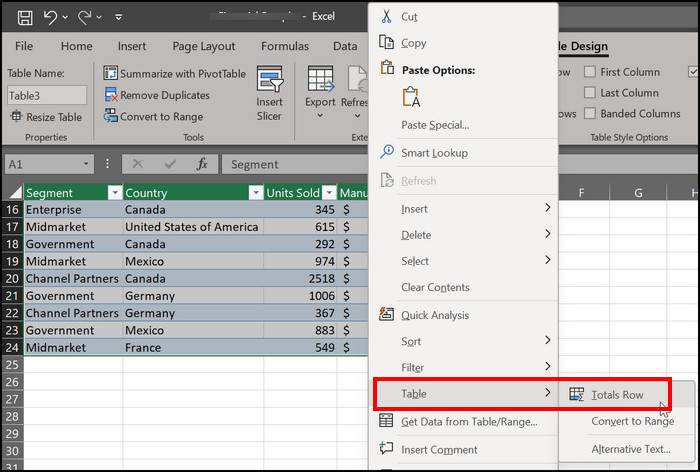Excel will insert a new row named Total at the end of the table. You can modify the row’s functionality after that.

Here’s a complete guide on how to Copy Values Without Formulas on Excel

### 3. Use a Formula to Add Totals Row

You simply can’t think of Excel’s practical cell modification without using a formula. Different functions and formulas are the ones that make Excel more flawless.

However, some users find the excel formula hard to implement. Since you’re here, there is no need to worry about that. Just follow my instructions to include a new formula to add total rows.

Here is the formula to add the totals row in Excel:

• Select the cell below the last cell of your table. For example, I have 17 cells available in this sheet. So, I clicked on the 18th.
• Type Total in that cell.
• Go to the formula bar and type in =SUBTOTAL(9, first cell: last cell).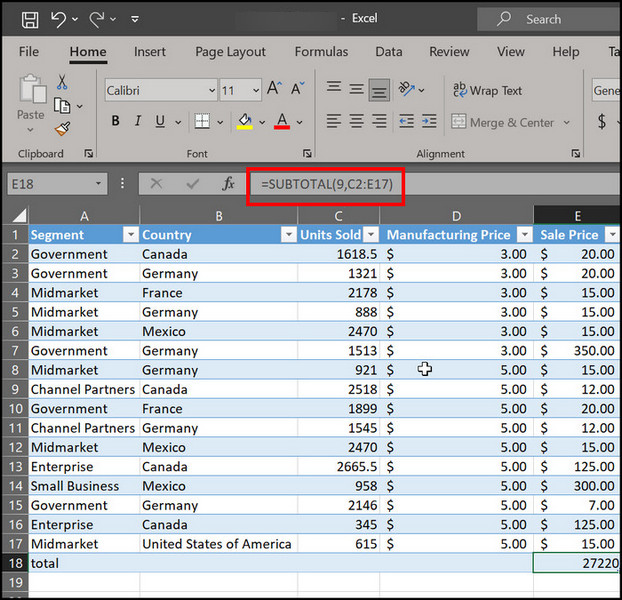• Press Enter to apply the formula.

Note: In this formula, 9 indicates you’ll sum up the selected cells. D2 is the first cell, and D16 is the last cell. So, the formula would be =Subtotal(9,C2:E17).

These are the most useful methods of adding a total in a row at the end of an Excel table. You can try any of these methods and get the same result.

Check out our separate post on Fix Microsoft Excel Freezing or Slow, Also, check out our separate post on how to MS Excel Lock a Cell

## How to Insert a Total Row in Excel on Mac

You can use the SUBTOTAL function to enable the Total row option on your Mac. As the Total Row default selections this function, you’ll be able to add hidden table rows in Excel. Follow further instructions to add the total row on Excel Mac.

Here’s the procedure to enable a total row in Excel Mac:

• Open Excel on your computer.
• Click on any cells of the table.
• Navigate to Table > click on Table Row.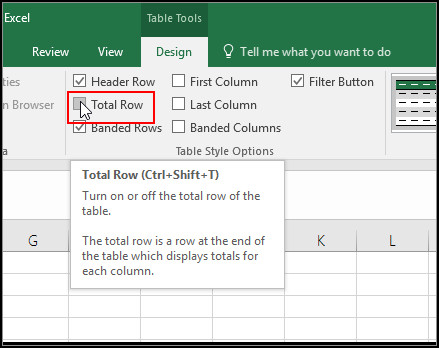You’ll find that a new row is included at the end of the table.

Now, if you have any further queries related to adding a total row, follow the subsequent section.

Follow our guide on how to Mail Merge in Outlook with Excel & Word, Here’s a complete guide on how to Lock and Unlock Cells in Excel

How do I add Total Row in an Excel Table?

Select the table where you want to include the additional row > Press Ctrl + Shift + Enter to add the row.

Can I Add an Extra row in Excel Web?

Yes, to add the row in Excel online, Click anywhere inside the table > Table Design tab > Style Options > Total Row.

Can I use a Formula to add the Totals row?

Using a formula is the safest method for inserting Totals rows in an Excel table.

## Wrapping Up

Creating an aggregate row at the end of an Excel table lets you summarize the column data without any delay. To sum up the rows, press Ctrl + Shift + T. Also, you can add the row from the Table Design tab.

Nevertheless, if you find any issues while following the instructions I’ve included here, just comment below. A group of Excel experts is ready to answer your queries 24/7.